Applications of Normal Distribution

Reasoning based on normal distributions is an important skill that goes throughout the rest of the course. In this lecture, we will look at a few problems that illustrate what you can do with normal distributions. One of the variables that we know do follow normal distributions is the height of people. For all these problems, we’re going to assume that women’s heights are normally distributed with a mean of 65 inches and a standard deviation of 3 inches. In the textbook’s notation, we can also state.# Finding Probabilities from Given Values of Random Variable

1)      What is the probability that a woman is between 64 inches and 69 inches tall (5’4” to 5’9”)? Put another way, what fraction of women’s heights are in this range? Using the notation of random variables, we would write this as P(64 < X < 69).

First, draw a horizontal axis and label it x, write the units (inches) below it, and draw a normal pdf centered over the mean of 65 inches. Then mark and label 65 on the axis, mark and label 64 to the left of it and 69 to the right of it, draw vertical lines from the 64 and the 69 to the curve and shade the part between them, above the x-axis, and under the curve: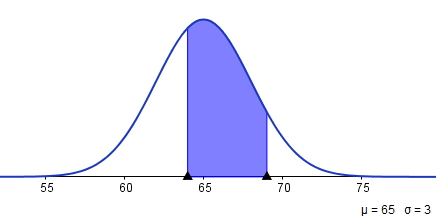If you are using GeoGebra, then you will immediately see that the software tells you P(64 < X <69) =0.5393. If you are using the calculator, then you need to find the normalcdf (not normalpdf) function. Enter the number on the left where the shading begins, the number on the right where it ends, the mean of the distribution, and its standard deviation, all separated by commas, normalcdf (64, 69, 65, 3), and you will get 0.539347. Round this to the nearest ten-thousandth (four places after the decimal point), or equivalently to the nearest hundredth of a percent, and you come up with the correct answer: 0.5393, or 53.93%.

In the last lecture, we mentioned that in the old days, everyone has to learn how to look up a Z-table, the table the shows the relationship between area and Z-score for the standard normal. Then how does GeoGebra and normalcdf do it? Well, it’s no magic. The software simply converts any normal distribution to a standard normal, using the familiar relationship of Z-score:So our example above will be converted to:, which gives you exactly the same area, just under a different scale. In your graphing calculator, this area can be found by using the command normalcdf (-0.33, 1.33, 0, 1).It’s not necessary that you always convert all normal distributions to Z, but it’s useful to recognize how it is handled by the software, since we will be doing the same later in inferential statistics.

2)      What is the probability that a woman is taller than 5 feet, 10 inches, or 70 inches? Put another way, what fraction of women are taller than 70 inches? This would be written as P(X > 70).

Start the same way as in Problem 1, but you have to mark and label only one number besides the mean, the 70. Then shade to the right of the 70, because that’s where the taller heights are: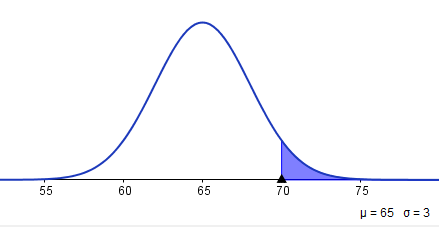GeoGebra is fairly self-explanatory here. With the calculator, the only complication using normalcdf is that there is no number on the right where the shading ends, so put in a big one, and if you’re not sure if it’s big enough put in a bigger one and see if it changes your answer, at least to the nearest ten-thousandth. normalcdf ( 70, 1000, 65, 3)=0.04779, so the rounded answer is 0.0478, or 4.78%.

# Find Cut-Off Values of the Random Variable from Probability

In the problems above, we found the probability that the random variable falls within a certain range. Now we’re going to reverse the process. We’ll start with the probability of a certain range, and then we’ll have to find the values of the random variable that determine that range. I’ll call these values cut-offs. Sometimes they are also called “inverse probability” problems.

In these three problems, we’ll use the same situation as above: Women’s heights are normally distributed with a mean of 65 inches and a standard deviation of 3 inches.

1)      How short does a woman have to be to be in the shortest 10% of women? If we call this cut-off c, this could be written as finding c such that P(X < c) = 0.10.

We’ll do the same kind of diagram as before, but this time we’ll label the known probability, 10%, and we do this above the shaded area, definitely not on the x-axis, because it’s an area, not a height. The hardest part of the diagram is deciding which side of the mean to put the c on and which side of the c to shade.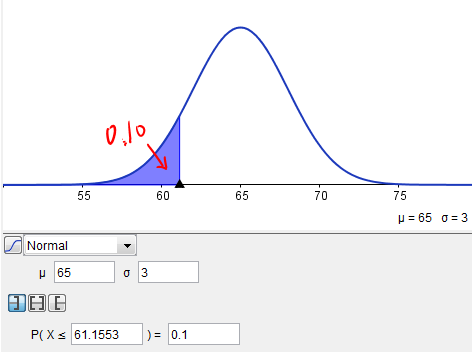You really have to think about it. In this case, since by definition 50% of women are shorter than the mean, the cut-off for 10% has to be less than the mean.

The picture here shows that how GeoGebra can be used to find the cut-off values: instead of entering the cut-off values, you can enter 0.10 as the probability, and GeoGebra will solve for the cut-off value (61.1553).

Using the calculator, you will need to resort to the invNorm function, followed by the percent of data under the normal curve to the left of (always to the left of, no matter which side of c the shading is on) the cut-off, then the mean and standard deviation, separated by commas.

So in our example, we will do invNorm (0.10, 65, 3), or, to the nearest inch, like the mean and standard deviation, 61 inches. So about 10% of women are shorter than 61 inches. You can check this using normalcdf, and you might as well use more of the cut-off than we rounded to, for greater assurance that your check shows you got the right answer. You get normalcdf (0, 61.1553, 65, 3), which come to 0.0999997, or 10%.

2)      How tall does a woman have to be to be in the tallest fourth of women? (What is the cut-off for the tallest 25% of women?) If we call this height c, we want to find the value of c such that P(X > c) = 0.25. Here’s the diagram: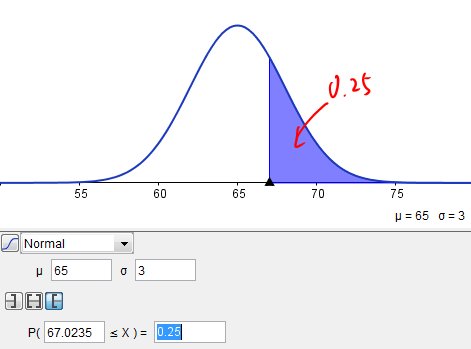In GeoGebra it’s quite simple: you will just have to switch the left to the right tail.

In the calculator, when we use invNorm we must put in 0.75, because the calculator finds cut-offs for areas to the left only: invNorm (0.75, 65, 3).  Here 0.75 comes from the fact that the total area must be equal to 1. When we subtract the area to the right, we are getting the area to the left of the cut-off.

Again, either GeoGebra or invNorm rely on the standard normal Z table to compute these values. To see how this is done, you will first need to first the cut-off value for the 25% area to the right: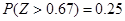On your graphing calculator, the result of 0.67 can be found by using invNorm (0.75, 0, 1). Then using the relationship between the Z score and X, we can solve for x as the unknown: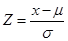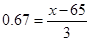Using the algebra you have learned, you will find x = 3*0.67 + 65 = 67.0, which is how the software arrived at the answer. You won’t have to do it this way every time, but it’s helpful to keep in mind, since this relation is used later on in finding the margin of error for confidence intervals.

3)      What if we’re interested in finding cut-offs for a middle group of women’s heights, say the middle 40%? Obviously, we’re looking for two numbers here, one on either side of the mean, with the same distance to the mean. Call them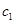and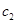. Then we are looking for these values so that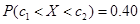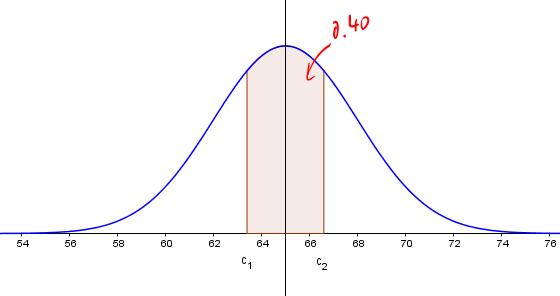You probably noticed that the normal calculator in GeoGebra can’t really find two cut-offs at oncein fact, the figure above was drawn using a different tool. Butandare not two independent values, since they are equally far from 65, the mean. To use the normal calculator, we must find out how much area is under the curve to the left of. Well, if 100% of area is under the entire curve, then what’s left over after taking away the middle 40% is 1-0.40=0.60, and since that 60% is split evenly between the two tails (the parts at the sides), that gives 30% for each tail. Sois the number such that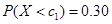.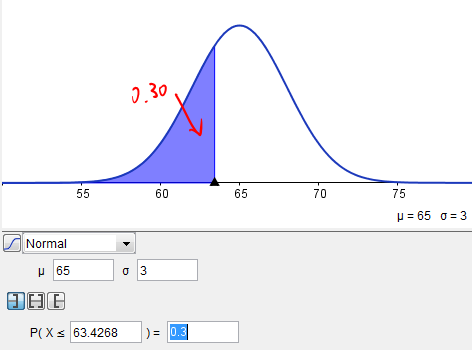So, the cut-off value on the left, is 63.4 inches.

How much area is there under the curve to the left of? Either subtract the 30% to the right from 100%, or add up the 30% in the left tail and the 40% in the middle, and you’ll get 70% either way. Sois the number such that, and you will find that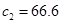inches. So to the first decimal, the middle 40% of heights go from 63.4 to 66.6 inches. If you use invNorm on a calculator, the two values can be found via invNorm(0.30, 65, 3), and invNorm(0.70, 65, 3), respectively.

# Summary

Here are a few tips that may help you solve problems related to the normal distribution:

1)      First identify the distribution: is it continuous? Is it Normal?

2)      Draw a graph of the normal PDF with the mean and standard deviation

3)      Examine the question to see whether you are looking for a probability, or cut-off values.

4)      Shade the approximate areas under the normal PDF.

5)      Use the software/calculator to solve the unknown, and compare the output with your graph.

Remember that if you have found a probability to be more than 1, then you should re-read the question to make sure you are solving the correct problem.